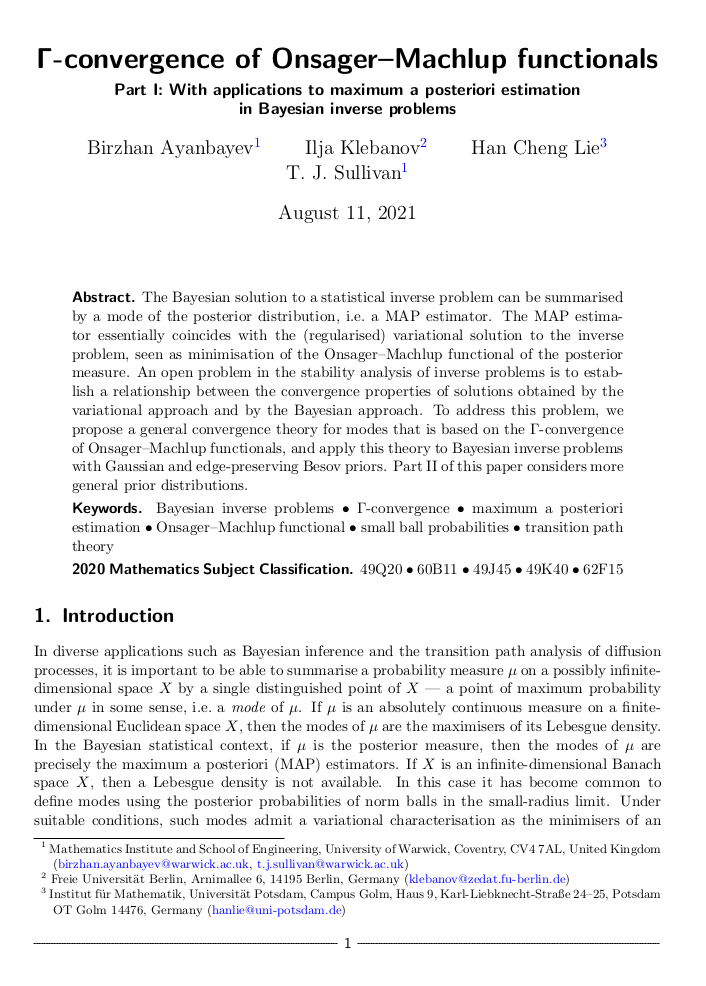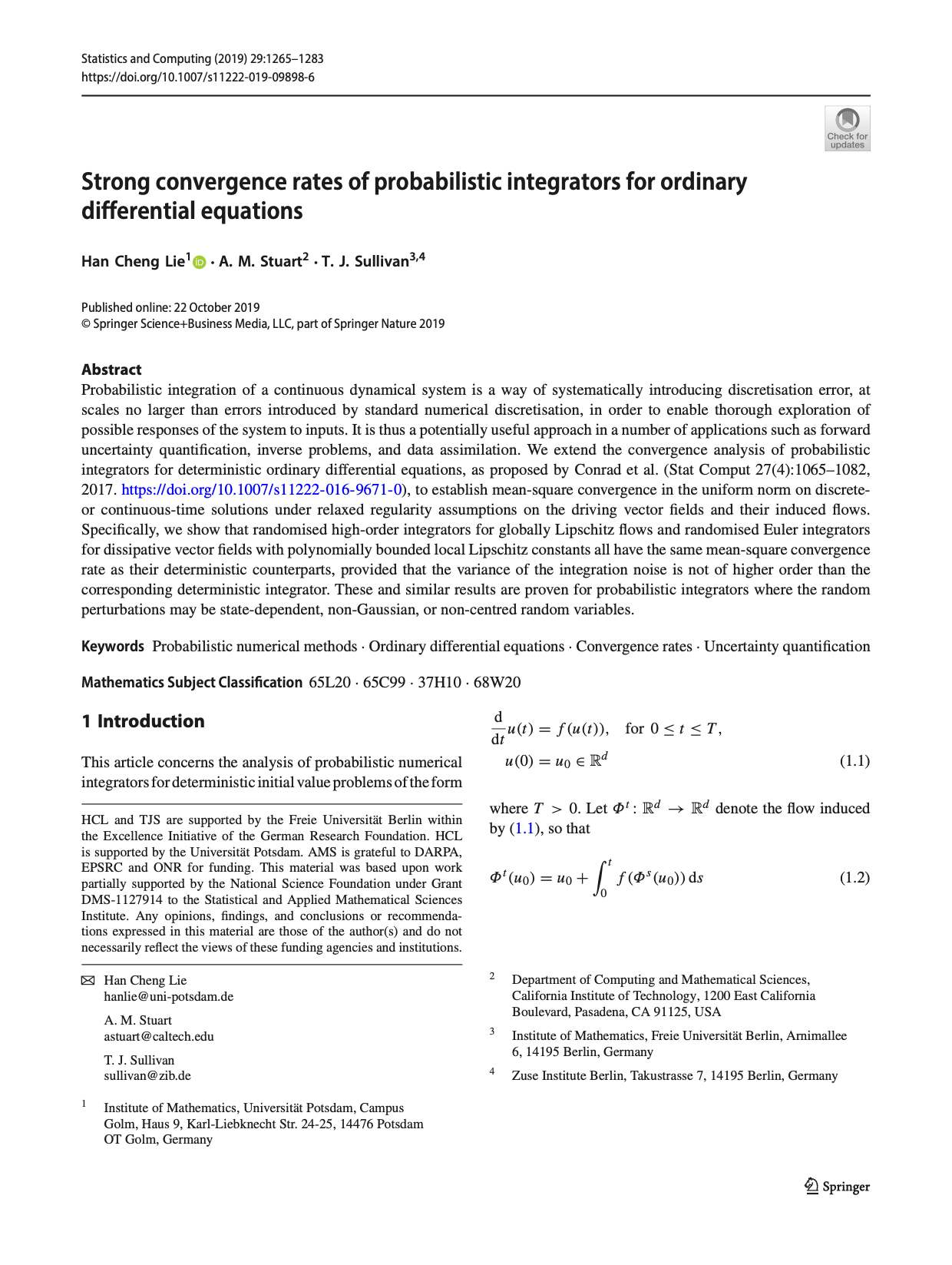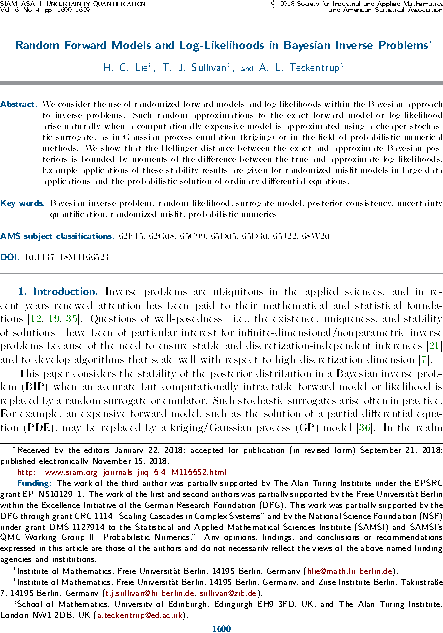# Tim Sullivan

### #lie

Clear Search### Γ-convergence of Onsager-Machlup functionals

Birzhan Ayanbayev, Ilja Klebanov, Han Cheng Lie, and I have just uploaded preprints of our work “Γ-convergence of Onsager–Machlup functionals” to the arXiv; this work consists of two parts, “Part I: With applications to maximum a posteriori estimation in Bayesian inverse problems” and “Part II: Infinite product measures on Banach spaces”.

The purpose of this work is to address a long-standing issue in the Bayesian approach to inverse problems, namely the joint stability of a Bayesian posterior and its modes (MAP estimators) when the prior, likelihood, and data are perturbed or approximated. We show that the correct way to approach this problem is to interpret MAP estimators as global weak modes in the sense of Helin and Burger (2015), which can be identified as the global minimisers of the Onsager–Machlup functional of the posterior distribution, and hence to provide a convergence theory for MAP estimators in terms of Γ-convergence of these Onsager–Machlup functionals. It turns out that posterior Γ-convergence can be assessed in a relatively straightforward manner in terms of prior Γ-convergence and continuous convergence of potentials (negative log-likelihoods). Over the two parts of the paper, we carry out this programme both in generality and for specific priors that are commonly used in Bayesian inverse problems, namely Gaussian and Besov priors (Lassas et al., 2009; Dashti et al., 2012).

Abstract (Part I). The Bayesian solution to a statistical inverse problem can be summarised by a mode of the posterior distribution, i.e. a MAP estimator. The MAP estimator essentially coincides with the (regularised) variational solution to the inverse problem, seen as minimisation of the Onsager–Machlup functional of the posterior measure. An open problem in the stability analysis of inverse problems is to establish a relationship between the convergence properties of solutions obtained by the variational approach and by the Bayesian approach. To address this problem, we propose a general convergence theory for modes that is based on the Γ-convergence of Onsager–Machlup functionals, and apply this theory to Bayesian inverse problems with Gaussian and edge-preserving Besov priors. Part II of this paper considers more general prior distributions.

Abstract (Part II). We derive Onsager–Machlup functionals for countable product measures on weighted $$\ell^{p}$$ subspaces of the sequence space $$\mathbb{R}^\mathbb{N}$$. Each measure in the product is a shifted and scaled copy of a reference probability measure on $$\mathbb{R}$$ that admits a sufficiently regular Lebesgue density. We study the equicoercivity and Γ-convergence of sequences of Onsager–Machlup functionals associated to convergent sequences of measures within this class. We use these results to establish analogous results for probability measures on separable Banach or Hilbert spaces, including Gaussian, Cauchy, and Besov measures with summability parameter $$1 \leq p \leq 2$$. Together with Part I of this paper, this provides a basis for analysis of the convergence of maximum a posteriori estimators in Bayesian inverse problems and most likely paths in transition path theory.

Published on Wednesday 11 August 2021 at 12:00 UTC #preprint #inverse-problems #modes #map-estimators #ayanbayev #klebanov #lie### Randomised integration for deterministic operator differential equations

Han Cheng Lie, Martin Stahn, and I have just uploaded a preprint of our recent work “Randomised one-step time integration methods for deterministic operator differential equations” to the arXiv. In this paper, we extend the analysis of Conrad et al. (2016) and Lie et al. (2019) to the case of evolutionary systems in Banach spaces or even Gel′fand triples, this being the right setting for many evolutionary partial differential equations.

Abstract. Uncertainty quantification plays an important role in applications that involve simulating ensembles of trajectories of dynamical systems. Conrad et al. (Stat. Comput., 2017) proposed randomisation of deterministic time integration methods as a strategy for quantifying uncertainty due to time discretisation. We consider this strategy for systems that are described by deterministic, possibly non-autonomous operator differential equations defined on a Banach space or a Gel′fand triple. We prove pathwise and expected error bounds on the random trajectories, given an assumption on the local truncation error of the underlying deterministic time integration and an assumption that the absolute moments of the random variables decay with the time step. Our analysis shows that the error analysis for differential equations in finite-dimensional Euclidean space carries over to infinite-dimensional settings.

Published on Wednesday 31 March 2021 at 09:00 UTC #preprint #prob-num #lie #stahn### Strong convergence rates of probabilistic integrators for ODEs in Statistics and Computing

The special issue of Statistics and Computing dedicated to probabilistic numerics carries the article “Strong convergence rates of probabilistic integrators for ODEs” by Han Cheng Lie, Andrew Stuart, and myself on the convergence analysis of randomised perturbation-based time-stepping methods for the solution of ODE initial value problems.

H. C. Lie, A. M. Stuart, and T. J. Sullivan. “Strong convergence rates of probabilistic integrators for ordinary differential equations.” Statistics and Computing 29(6):1265–1283, 2019. doi:10.1007/s11222-019-09898-6

Abstract. Probabilistic integration of a continuous dynamical system is a way of systematically introducing discretisation error, at scales no larger than errors introduced by standard numerical discretisation, in order to enable thorough exploration of possible responses of the system to inputs. It is thus a potentially useful approach in a number of applications such as forward uncertainty quantification, inverse problems, and data assimilation. We extend the convergence analysis of probabilistic integrators for deterministic ordinary differential equations, as proposed by Conrad et al. (2016), to establish mean-square convergence in the uniform norm on discrete- or continuous-time solutions under relaxed regularity assumptions on the driving vector fields and their induced flows. Specifically, we show that randomised high-order integrators for globally Lipschitz flows and randomised Euler integrators for dissipative vector fields with polynomially bounded local Lipschitz constants all have the same mean-square convergence rate as their deterministic counterparts, provided that the variance of the integration noise is not of higher order than the corresponding deterministic integrator. These and similar results are proven for probabilistic integrators where the random perturbations may be state-dependent, non-Gaussian, or non-centred random variables.

Published on Tuesday 5 November 2019 at 12:00 UTC #publication #stco #prob-num #lie #stuart### Implicit Probabilistic Integrators in NeurIPS

The paper “Implicit probabilistic integrators for ODEs” by Onur Teymur, Han Cheng Lie, Ben Calderhead and myself has now appeared in Advances in Neural Information Processing Systems 31 (NeurIPS 2018). This paper forms part of an expanding body of work that provides mathematical convergence analysis of probabilistic solvers for initial value problems, in this case implicit methods such as (probabilistic versions of) the multistep Adams–Moulton method.

O. Teymur, H. C. Lie, T. J. Sullivan, and B. Calderhead. “Implicit probabilistic integrators for ODEs” in Advances in Neural Information Processing Systems 31 (NIPS 2018), ed. S. Bengio, H. Wallach, H. Larochelle, K. Grauman, N. Cesa-Bianchi, and R. Garnett. 7244–7253, 2018. http://papers.nips.cc/paper/7955-implicit-probabilistic-integrators-for-odes

Abstract. We introduce a family of implicit probabilistic integrators for initial value problems (IVPs), taking as a starting point the multistep Adams–Moulton method. The implicit construction allows for dynamic feedback from the forthcoming time-step, in contrast to previous probabilistic integrators, all of which are based on explicit methods. We begin with a concise survey of the rapidly-expanding field of probabilistic ODE solvers. We then introduce our method, which builds on and adapts the work of Conrad et al. (2016) and Teymur et al. (2016), and provide a rigorous proof of its well-definedness and convergence. We discuss the problem of the calibration of such integrators and suggest one approach. We give an illustrative example highlighting the effect of the use of probabilistic integrators — including our new method — in the setting of parameter inference within an inverse problem.

Published on Thursday 13 December 2018 at 12:00 UTC #publication #nips #neurips #prob-num #lie #teymur #calderhead### Random Bayesian inverse problems in JUQ

The article “Random forward models and log-likelihoods in Bayesian inverse problems” by Han Cheng Lie, Aretha Teckentrup, and myself has now appeared in its final form in the SIAM/ASA Journal on Uncertainty Quantification, volume 6, issue 4. This paper considers the effect of approximating the likelihood in a Bayesian inverse problem by a random surrogate, as frequently happens in applications, with the aim of showing that the perturbed posterior distribution is close to the exact one in a suitable sense. This article considers general randomisation models, and thereby expands upon the previous investigations of Stuart and Teckentrup (2018) in the Gaussian setting.

H. C. Lie, T. J. Sullivan, and A. L. Teckentrup. “Random forward models and log-likelihoods in Bayesian inverse problems.” SIAM/ASA Journal on Uncertainty Quantification 6(4):1600–1629, 2018. doi:10.1137/18M1166523

Abstract. We consider the use of randomised forward models and log-likelihoods within the Bayesian approach to inverse problems. Such random approximations to the exact forward model or log-likelihood arise naturally when a computationally expensive model is approximated using a cheaper stochastic surrogate, as in Gaussian process emulation (kriging), or in the field of probabilistic numerical methods. We show that the Hellinger distance between the exact and approximate Bayesian posteriors is bounded by moments of the difference between the true and approximate log-likelihoods. Example applications of these stability results are given for randomised misfit models in large data applications and the probabilistic solution of ordinary differential equations.

Published on Monday 10 December 2018 at 12:00 UTC #publication #bayesian #inverse-problems #juq #prob-num #lie #teckentrup